# NCERT Solutions: Classification of Elements & Periodicity in Properties Notes | Study NCERTs for NEET: Textbooks, Tests & Solutions - NEET

## NEET: NCERT Solutions: Classification of Elements & Periodicity in Properties Notes | Study NCERTs for NEET: Textbooks, Tests & Solutions - NEET

The document NCERT Solutions: Classification of Elements & Periodicity in Properties Notes | Study NCERTs for NEET: Textbooks, Tests & Solutions - NEET is a part of the NEET Course NCERTs for NEET: Textbooks, Tests & Solutions.
All you need of NEET at this link: NEET

Classification of Elements & Periodicity in Properties

Ques 3.1: What is the basic theme of organisation in the periodic table?
Ans:- The basic theme of organisation of elements in the periodic table is to classify the elements in periods and groups according to their properties. This arrangement makes the study of elements and their compounds simple and systematic. In the periodic table, elements with similar properties are placed in the same group.

Ques 3.2: Which important property did Mendeleev use to classify the elements in his periodic table and did he stick to that?
Ans:- Mendeleev arranged the elements in his periodic table ordered by atomic weight or mass. He arranged the elements in periods and groups in order of their increasing atomic weight. He placed the elements with similar properties in the same group.

However, he did not stick to this arrangement for long. He found out that if the elements were arranged strictly in order of their increasing atomic weights, then some elements did not fit within this scheme of classification.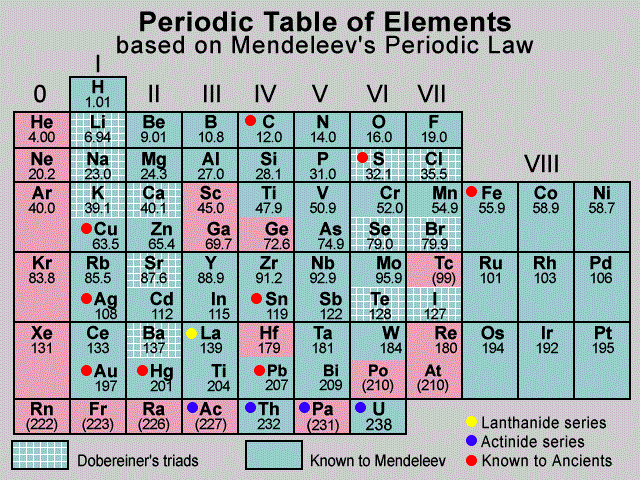Therefore, he ignored the order of atomic weights in some cases. For example, the atomic weight of iodine is lower than that of tellurium. Still Mendeleev placed tellurium (in Group VI) before iodine (in Group VII) simply because iodine’s properties are so similar to fluorine, chlorine, and bromine.

Ques 3.3: What is the basic difference in approach between the Mendeleev’s Periodic Law and the Modern Periodic Law?
Ans:- Mendeleev’s Periodic Law states that the physical and chemical properties of elements are periodic functions of their atomic weights. On the other hand, the Modern periodic Law states that the physical and chemical properties of elements are periodic functions of their atomic numbers.

Ques 3.4: On the basis of quantum numbers, justify that the sixth period of the periodic table should have 32 elements.
Ans:- In the periodic table of the elements, a period indicates the value of the principal quantum number (n) for the outermost shells. Each period begins with the filling of principal quantum number (n). The value of n for the sixth period is 6. For n = 6, azimuthal quantum number (l) can have values of 0, 1, 2, 3, 4.

According to Aufbau’s principle, electrons are added to different orbitals in order of their increasing energies. The energy of the 6d subshell is even higher than that of the 7s subshell.

In the 6th period, electrons can be filled in only 6s, 4f, 5d, and 6 p subshells. Now, 6s has one orbital, 4f has seven orbitals, 5d has five orbitals, and 6has three orbitals. Therefore, there are a total of sixteen (1 + 7 + 5 + 3 = 16) orbitals available. According to Pauli’s exclusion principle, each orbital can accommodate a maximum of 2 electrons. Thus, 16 orbitals can accommodate a maximum of 32 electrons.

Hence, the sixth period of the periodic table should have 32 elements.

Ques 3.5: In terms of period and group where would you locate the element with =114?
Ans:- Elements with atomic numbers from Z = 87 to Z = 114 are present in the 7th period of the periodic table. Thus, the element with Z = 114 is present in the 7th period of the periodic table.

In the 7th period, first two elements with Z = 87 and Z= 88 are s-block elements, the next 14 elements excluding = 89 i.e., those with Z = 90 – 103 are f – block elements, ten elements with Z = 89 and Z = 104 – 112 are d – block elements, and the elements with = 113 – 118 are p – block elements. Therefore, the element with Z = 114 is the second p – block element in the 7th period.

Thus, the element with Z = 114 is present in the 7th period and 4th group of the periodic table.

Ques 3.6: Write the atomic number of the element present in the third period and seventeenth group of the periodic table.
Ans:- There are two elements in the 1st period and eight elements in the 2nd period., The third period starts with the element with = 11. Now, there are eight elements in the third period. Thus, the 3rd period ends with the element with Z=18 i.e., the element in the 18th group of the third period has Z =18. Hence, the element in the 17th group of the third period has atomic number Z = 17.

Ques 3.7: Which element do you think would have been named by
(i) Lawrence Berkeley Laboratory
(ii) Seaborg’s group?
Ans:-
(i) Lawrencium (Lr) with Z = 103 and Berkelium (Bk) with Z = 97
(ii) Seaborgium (Sg) with Z = 106

Ques 3.8: Why do elements in the same group have similar physical and chemical properties?
Ans:- The physical and chemical properties of elements depend on the number of valence electrons. Elements present in the same group have the same number of valence electrons. Therefore, elements present in the same group have similar physical and chemical properties.

Ques 3.9: What does atomic radius and ionic radius really mean to you?
Ans:- Atomic radius is the radius of an atom. It measures the size of an atom. If the element is a metal, then the atomic radius refers to the metallic radius, and if the element is a non-metal, then it refers to the covalent radius. Metallic radius is calculated as half the internuclear distance separating the metal cores in the metallic crystal. For example, the internuclear distance between two adjacent copper atoms in solid copper is 256 pm. Thus, the metallic radius of copper is taken as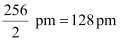.

Covalent radius is measured as the distance between two atoms when they are found together by a single bond in a covalent molecule. For example, the distance between two chlorine atoms in chlorine molecule is 198 pm. Thus, the covalent radius of chlorine is taken as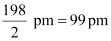.

Ionic radius means the radius of an ion (cation or anion). The ionic radii can be calculated by measuring the distances between the cations and anions in ionic crystals.

Since a cation is formed by removing an electron from an atom, the cation has fewer electrons than the parent atom resulting in an increase in the effective nuclear charge. Thus, a cation is smaller than the parent atom. For example, the ionic radius of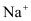ion is 95 pm, whereas the atomic radius of Na atom is 186 pm. On the other hand, an anion is larger in size than its parent atom. This is because an anion has the same nuclear charge, but more electrons than the parent atom resulting in an increased repulsion among the electrons and a decrease in the effective nuclear charge. For example, the ionic radius of F ion is 136 pm, whereas the atomic radius of F atom is 64 pm.

Ques 3.10: How does atomic radius vary in a period and in a group? How do you explain the variation?
Ans:- Atomic radius generally decreases from left to right across a period. This is because within a period, the outer electrons are present in the same valence shell and the atomic number increases from left to right across a period, resulting in an increased effective nuclear charge. As a result, the attraction of electrons to the nucleus increases.

On the other hand, the atomic radius generally increases down a group. This is because down a group, the principal quantum number (n) increases which results in an increase of the distance between the nucleus and valence electrons.

Ques 3.11: What do you understand by isoelectronic species? Name a species that will be isoelectronic with each of the following atoms or ions.
(i) F–
(ii) Ar
(iii) Mg2+
(iv) Rb+
Ans:- Atoms and ions having the same number of electrons are called isoelectronic species.

(i) F– ion has 9 + 1 = 10 electrons. Thus, the species isoelectronic with it will also have 10 electrons. Some of its isoelectronic species are Na  ion (11 – 1 = 10 electrons), Ne (10 electrons), O2– ion (8 + 2 = 10 electrons), and Al3+  ion (13 – 3 = 10 electrons).

(ii) Ar has 18 electrons. Thus, the species isoelectronic with it will also have 18 electrons. Some of its isoelectronic species are S2– ion (16+2 = 18 electrons), Cl– ion (17 + 1 = 18 electrons), K  ion (19 – 1 = 18 electrons), and Ca2+  ion (20 – 2 = 18 electrons).

(iii) Mg2+  ion has 12 – 2 = 10 electrons. Thus, the species isoelectronic with it will also have 10 electrons. Some of its isoelectronic species are F– ion (9 +1 = 10 electrons), Ne (10 electrons), O2– ion (8 + 2 = 10 electrons), and Al3+  ion (13 – 3 = 10 electrons).

(iv) Rb+  ion has 37 – 1 = 36 electrons. Thus, the species isoelectronic with it will also have 36 electrons. Some of its isoelectronic species are Br ion (35 + 1 = 36 electrons), Kr (36 electrons), and Sr2+  ion (38 – 2 = 36 electrons).
Ques 3.12: Consider the following species:
N3–, O2–, F, Na+ , Mg2+  and Al3+
(a) What is common in them?
(b) Arrange them in the order of increasing ionic radii.
Ans:- (a) Each of the given species (ions) has the same number of electrons (10 electrons). Hence, the given species are isoelectronic.

(b) The ionic radii of isoelectronic species increases with a decrease in the magnitudes of nuclear charge.

The arrangement of the given species in order of their increasing nuclear charge is as follows:

N3– < O2– < F < Na+  < Mg2+  < Al3+

Nuclear charge = +7 +  8  +9  +11  +12  +13

Therefore, the arrangement of the given species in order of their increasing ionic radii is as follows:

Al3+  < Mg2+ < Na+  < F < O2– < N3–

Ques 3.13: Explain why cations are smaller and anions larger in radii than their parent atoms?
Ans:- A cation has a fewer number of electrons than its parent atom, while its nuclear charge remains the same. As a result, the attraction of electrons to the nucleus is more in a cation than in its parent atom. Therefore, a cation is smaller in size than its parent atom.

On the other hand, an anion has one or more electrons than its parent atom, resulting in an increased repulsion among the electrons and a decrease in the effective nuclear charge. As a result, the distance between the valence electrons and the nucleus is more in anions than in it’s the parent atom. Hence, an anion is larger in radius than its parent atom.

Ques 3.14: What is the significance of the terms - ‘isolated gaseous atom’ and ‘ground state’ while defining the ionization enthalpy and electron gain enthalpy?
Hint: Requirements for comparison purposes.
Ans:- Ionization enthalpy is the energy required to remove an electron from an isolated gaseous atom in its ground state. Although the atoms are widely separated in the gaseous state, there are some amounts of attractive forces among the atoms. To determine the ionization enthalpy, it is impossible to isolate a single atom. But, the force of attraction can be further reduced by lowering the pressure. For this reason, the term ‘isolated gaseous atom’ is used in the definition of ionization enthalpy.

Ground state of an atom refers to the most stable state of an atom. If an isolated gaseous atom is in its ground state, then less amount energy would be required to remove an electron from it. Therefore, for comparison purposes, ionization enthalpy and electron gain enthalpy must be determined for an ‘isolated gaseous atom’ and its ‘ground state’.

Ques 3.15: Energy of an electron in the ground state of the hydrogen atom is –2.18 × 10–18 J. Calculate the ionization enthalpy of atomic hydrogen in terms of J mol–1.
Ans:- It is given that the energy of an electron in the ground state of the hydrogen atom is –2.18 ×10–18 J.

Therefore, the energy required to remove that electron from the ground state of hydrogen atom is 2.18 × 10–18 J.Ionization enthalpy of atomic hydrogen = 2.18 × 10–18 J

Hence, ionization enthalpy of atomic hydrogen in terms of J mol–1 = 2.18 × 10–18 × 6.02 × 1023 J mol–1 = 1.31 × 106 J mol–1

Ques 3.16: Among the second period elements the actual ionization enthalpies are in the order
Li < B < Be < C < O < N < F < Ne.
Explain why
(i) Be has higher Δithan B
(ii) O has lower Δithan N and F?
Ans:- (i) During the process of ionization, the electron to be removed from beryllium atom is a 2s-electron, whereas the electron to be removed from boron atom is a 2p­-electron. Now, 2s-electrons are more strongly attached to the nucleus than 2p-electrons. Therefore, more energy is required to remove a 2-electron of beryllium than that required to remove a 2p-electron of boron. Hence, beryllium has higher ΔiH than boron.

(ii) In nitrogen, the three 2p-electrons of nitrogen occupy three different atomic orbitals. However, in oxygen, two of the four 2p-electrons of oxygen occupy the same 2p-orbital. This results in increased electron-electron repulsion in oxygen atom. As a result, the energy required to remove the fourth 2p-electron from oxygen is less as compared to the energy required to remove one of the three 2p-electrons from nitrogen. Hence, oxygen has lower ΔiH than nitrogen.

Fluorine contains one electron and one proton more than oxygen. As the electron is being added to the same shell, the increase in nuclear attraction (due to the addition of a proton) is more than the increase in electronic repulsion (due to the addition of an electron). Therefore, the valence electrons in fluorine atom experience a more effective nuclear charge than that experienced by the electrons present in oxygen. As a result, more energy is required to remove an electron from fluorine atom than that required to remove an electron from oxygen atom. Hence, oxygen has lower ΔiH than fluorine.

Ques 3.17: How would you explain the fact that the first ionization enthalpy of sodium is lower than that of magnesium but its second ionization enthalpy is higher than that of magnesium?
Ans:- The first ionization enthalpy of sodium is more than that of magnesium. This is primarily because of two reasons:

1. The atomic size of sodium is greater than that of magnesium
2. The effective nuclear charge of magnesium is higher than that of sodium

For these reasons, the energy required to remove an electron from magnesium is more than the energy required in sodium. Hence, the first ionization enthalpy of sodium is lower than that of magnesium.

However, the second ionization enthalpy of sodium is higher than that of magnesium. This is because after losing an electron, sodium attains the stable noble gas configuration. On the other hand, magnesium, after losing an electron still has one electron in the 3s-orbital. In order to attain the stable noble gas configuration, it still has to lose one more electron. Thus, the energy required to remove the second electron in case of sodium is much higher than that required in case of magnesium. Hence, the second ionization enthalpy of sodium is higher than that of magnesium.

Ques 3.18: What are the various factors due to which the ionization enthalpy of the main group elements tends to decrease down a group?
Ans:- The factors responsible for the ionization enthalpy of the main group elements to decrease down a group are listed below:

(i) Increase in the atomic size of elements: As we move down a group, the number of shells increases. As a result, the atomic size also increases gradually on moving down a group. As the distance of the valence electrons from the nucleus increases, the electrons are not held very strongly. Thus, they can be removed easily. Hence, on moving down a group, ionization energy decreases.

(ii) Increase in the shielding effect: The number of inner shells of electrons increases on moving down a group. Therefore, the shielding of the valence electrons from the nucleus by the inner core electrons increases down a group. As a result, the valence electrons are not held very tightly by the nucleus. Hence, the energy required to remove a valence electron decreases down a group.

Ques 3.19:
The first ionization enthalpy values (in kJmol–1) of group 13 elements are :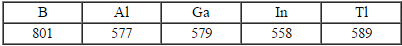How would you explain this deviation from the general trend?
Ans:- On moving down a group, ionization enthalpy generally decreases due to an increase in the atomic size and shielding. Thus, on moving down group 13, ionization enthalpy decreases from B to Al. But, Ga has higher ionization enthalpy than Al. Al follows immediately after s–block elements, whereas Ga follows after d–block elements. The shielding provided by d-electrons is not very effective. These electrons do not shield the valence electrons very effectively. As a result, the valence electrons of Ga experience a greater effective nuclear charge than those of Al. Further, moving from Ga to In, the ionization enthalpy decreases due to an increase in the atomic size and shielding. But, on moving from In to Tl, the ionization enthalpy again increases. In the periodic table, Tl follows after 4f and 5d electrons. The shielding provided by the electrons in both these orbitals is not very effective. Therefore, the valence electron is held quite strongly by the nucleus. Hence, the ionization energy of Tl is on the higher side.

Ques 3.20: Which of the following pairs of elements would have a more negative electron gain enthalpy?
(i) O or F
(ii) F or Cl
Ans:- (i) O and F are present in the same period of the periodic table. An F atom has one proton and one electron more than O and as an electron is being added to the same shell, the atomic size of F is smaller than that of O. As F contains one proton more than O, its nucleus can attract the incoming electron more strongly in comparison to the nucleus of O atom. Also, F needs only one more electron to attain the stable noble gas configuration. Hence, the electron gain enthalpy of F is more negative than that of O.

(ii) F and Cl belong to the same group of the periodic table. The electron gain enthalpy usually becomes less negative on moving down a group. However, in this case, the value of the electron gain enthalpy of Cl is more negative than that of F. This is because the atomic size of F is smaller than that of Cl. In F, the electron will be added to quantum level n = 2, but in Cl, the electron is added to quantum level n = 3. Therefore, there are less electron- electron repulsions in Cl and an additional electron can be accommodated easily. Hence, the electron gain enthalpy of Cl is more negative than that of F.

Ques 3.21: Would you expect the second electron gain enthalpy of O as positive, more negative or less negative than the first? Justify your answer.
Ans:- When an electron is added to O atom to form O ion, energy is released. Thus, the first electron gain enthalpy of O is negative.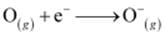On the other hand, when an electron is added to O ion to form O2– ion, energy has to be given out in order to overcome the strong electronic repulsions. Thus, the second electron gain enthalpy of O is positive.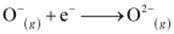Ques 3.22: What is the basic difference between the terms electron gain enthalpy and electronegativity?
Ans:- Electron gain enthalpy is the measure of the tendency of an isolated gaseous atom to accept an electron, whereas electronegativity is the measure of the tendency of an atom in a chemical compound to attract a shared pair of electrons.

Ques 3.23: How would you react to the statement that the electronegativity of N on Pauling scale is 3.0 in all the nitrogen compounds?
Ans:- Electronegativity of an element is a variable property. It is different in different compounds. Hence, the statement which says that the electronegativity of N on Pauling scale is 3.0 in all nitrogen compounds is incorrect. The electronegativity of N is different in NH3 and NO2.

Ques 3.24: Describe the theory associated with the radius of an atom as it
(a) gains an electron
(b) loses an electron
Ans:-  (a) When an atom gains an electron, its size increases. When an electron is added, the number of electrons goes up by one. This results in an increase in repulsion among the electrons. However, the number of protons remains the same. As a result, the effective nuclear charge of the atom decreases and the radius of the atom increases.

(b) When an atom loses an electron, the number of electrons decreases by one while the nuclear charge remains the same. Therefore, the interelectronic repulsions in the atom decrease. As a result, the effective nuclear charge increases. Hence, the radius of the atom decreases.

Ques 3.25: Would you expect the first ionization enthalpies for two isotopes of the same element to be the same or different? Justify your answer.
Ans:- The ionization enthalpy of an atom depends on the number of electrons and protons (nuclear charge) of that atom. Now, the isotopes of an element have the same number of protons and electrons. Therefore, the first ionization enthalpy for two isotopes of the same element should be the same.

Ques 3.26: What are the major differences between metals and non-metals?Ans:-

 Metals Non–metals 1. Metals can lose electrons easily. 1. Non-metals cannot lose electrons easily. 2. Metals cannot gain electrons easily. 2. Non-metals can gain electrons easily. 3. Metals generally form ionic compounds. 3. Non–metals generally form covalent compounds. 4. Metals oxides are basic in nature. 4. Non–metallic oxides are acidic in nature. 5. Metals have low ionization enthalpies. 5. Non–metals have high ionization enthalpies. 6. Metals have less negative electron gain enthalpies. 6. Non­–metals have high negative electron gain enthalpies. 7. Metals are less electronegative. They are rather electropositive elements. 7. Non–metals are electronegative. 8. Metals have a high reducing power. 8. Non–metals have a low reducing power.

Ques 3.27: Use the periodic table to answer the following questions.
(a) Identify an element with five electrons in the outer subshell.
(b) Identify an element that would tend to lose two electrons.
(c) Identify an element that would tend to gain two electrons.
(d) Identify the group having metal, non-metal, liquid as well as gas at the room temperature.
Ans:-  (a) The electronic configuration of an element having 5 electrons in its outermost subshell should be ns2 np5. This is the electronic configuration of the halogen group. Thus, the element can be F, CL, Br, I, or At.

(b) An element having two valence electrons will lose two electrons easily to attain the stable noble gas configuration. The general electronic configuration of such an element will be ns2. This is the electronic configuration of group 2 elements. The elements present in group 2 are Be, Mg, Ca, Sr, Ba.

(c) An element is likely to gain two electrons if it needs only two electrons to attain the stable noble gas configuration. Thus, the general electronic configuration of such an element should be ns2 np4. This is the electronic configuration of the oxygen family.

(d) Group 17 has metal, non–metal, liquid as well as gas at room temperature.

Ques 3.28: The increasing order of reactivity among group 1 elements is Li < Na < K < Rb <Cs whereas that among group 17 elements is F > CI > Br > I. Explain.
Ans:- The elements present in group 1 have only 1 valence electron, which they tend to lose. Group 17 elements, on the other hand, need only one electron to attain the noble gas configuration. On moving down group 1, the ionization enthalpies decrease. This means that the energy required to lose the valence electron decreases. Thus, reactivity increases on moving down a group. Thus, the increasing order of reactivity among group 1 elements is as follows:

Li < Na < K < Rb < Cs

In group 17, as we move down the group from Cl to I, the electron gain enthalpy becomes less negative i.e., its tendency to gain electrons decreases down group 17. Thus, reactivity decreases down a group. The electron gain enthalpy of F is less negative than Cl. Still, it is the most reactive halogen. This is because of its low bond dissociation energy. Thus, the decreasing order of reactivity among group 17 elements is as follows:

F > Cl > Br > I

Ques 3.29: Write the general outer electronic configuration of s-, p-, d- and f- block elements.
Ans:- Element General outer electronic configuration
s–block :   ns1–2, where n = 2 – 7
p–block:   ns2np1–6, where n = 2 – 6
d–block:  (n–1) d1–10 ns0–2, where n = 4 – 7
f–block:   (n–2)f1–14(n–1)d0–10ns2, where n = 6 – 7

Ques 3.30: Assign the position of the element having outer electronic configuration
(i) ns2 np4 for = 3
(ii) (- 1)d2 ns2 for = 4, and
(iii) (n - 2) f7 (n - 1)d1 ns2 for = 6, in the periodic table.
Ans:-  (i) Since n = 3, the element belongs to the 3rd period. It is a p–block element since the last electron occupies the p–orbital.

There are four electrons in the p–orbital. Thus, the corresponding group of the element
= Number of s–block groups number of d–block groups number of p–electrons
= 2 +10 + 4
= 16

Therefore, the element belongs to the 3rd period and 16th group of the periodic table. Hence, the element is Sulphur.

(ii) Since n = 4, the element belongs to the 4th period. It is a d–block element as d–orbitals are incompletely filled.
There are 2 electrons in the d–orbital.
Thus, the corresponding group of the element
= Number of s–block groups number of d–block groups
= 2 + 2
= 4

Therefore, it is a 4th period and 4th group element. Hence, the element is Titanium.

(iii) Since n = 6, the element is present in the 6th period. It is an f –block element as the last electron occupies the f–orbital. It belongs to group 3 of the periodic table since all f-block elements belong to group 3. Its electronic configuration is [Xe] 4f7 5d1 6s2. Thus, its atomic number is 54 + 7 + 2 + 1 = 64. Hence, the element is Gadolinium.

Ques 3.31: The first (ΔiH1) and the second (ΔiH) ionization enthalpies (in kJ mol–1) and the (ΔegH) electron gain enthalpy (in kJ mol–1) of a few elements are given below:

 Elements ΔiH ΔiH ΔegH I 520 7300 –60 II 419 3051 –48 III 1681 3374 –328 IV 1008 1846 –295 V 2372 5251 +48 VI 738 1451 –40

Which of the above elements is likely to be:
(a) the least reactive element.
(b) the most reactive metal.
(c) the most reactive non-metal.
(d) the least reactive non-metal.
(e) the metal which can form a stable binary halide of the formula MX2, (X=halogen).
(f) the metal which can form a predominantly stable covalent halide of the formula MX (X=halogen)?
Ans:-  (a) Element V is likely to be the least reactive element. This is because it has the highest first ionization enthalpy (ΔiH1) and a positive electron gain enthalpy (ΔegH).

(b) Element II is likely to be the most reactive metal as it has the lowest first ionization enthalpy (ΔiH1) and a low negative electron gain enthalpy (ΔegH).

(c) Element III is likely to be the most reactive non–metal as it has a high first ionization enthalpy (ΔiH1) and the highest negative electron gain enthalpy (ΔegH).

(d) Element V is likely to be the least reactive non–metal since it has a very high first ionization enthalpy (ΔiH2) and a positive electron gain enthalpy (ΔegH).

(e) Element VI has a low negative electron gain enthalpy (ΔegH). Thus, it is a metal. Further, it has the lowest second ionization enthalpy (ΔiH2). Hence, it can form a stable binary halide of the formula MX2 (X=halogen).

(f) Element I has low first ionization energy and high second ionization energy. Therefore, it can form a predominantly stable covalent halide of the formula MX (X=halogen).

Ques 3.32: Predict the formula of the stable binary compounds that would be formed by the combination of the following pairs of elements.
(a) Lithium and oxygen
(b) Magnesium and nitrogen
(c) Aluminium and iodine
(d) Silicon and oxygen
(e) Phosphorus and fluorine
(f) Element 71 and fluorine
Ans:-  (a) LiO2
(b) Mg3N2
(c) AlI3
(d) SiO2
(e) PF3 or PF5
(f) The element with the atomic number 71 is Lutetium (Lu). It has valency 3. Hence, the formula of the compound is LuF3.

Ques 3.33: In the modern periodic table, the period indicates the value of:
(a) Atomic number
(b) Atomic mass
(c) Principal quantum number
(d) Azimuthal quantum number.
Ans:- The value of the principal quantum number (n) for the outermost shell or the valence shell indicates a period in the Modern periodic table.

Ques 3.34: Which of the following statements related to the modern periodic table is incorrect?

(a) The p-block has 6 columns, because a maximum of 6 electrons can occupy all the orbitals in a p-shell.

(b) The d-block has 8 columns, because a maximum of 8 electrons can occupy all the orbitals in a d-subshell.

(c) Each block contains a number of columns equal to the number of electrons that can occupy that subshell.

(d) The block indicates value of azimuthal quantum number () for the last subshell that received electrons in building up the electronic configuration.
Ans:- The d-block has 10 columns because a maximum of 10 electrons can occupy all the orbitals in a d subshell.

Ques 3.35:Anything that influences the valence electrons will affect the chemistry of the element. Which one of the following factors does not affect the valence shell?
(a) Valence principal quantum number (n)
(b) Nuclear charge (Z)
(c) Nuclear mass
(d) Number of core electrons.

Ans:- Nuclear mass does not affect the valence electrons

Ques 3.36: The size of isoelectronic species — F, Ne and Na+  is affected by
(a) Nuclear charge ()
(b) Valence principal quantum number (n)
(c) Electron-electron interaction in the outer orbitals
(d) None of the factors because their size is the same.
Ans:- The size of an isoelectronic species increases with a decrease in the nuclear charge (Z). For example, the order of the increasing nuclear charge of F, Ne, and Na  is as follows:

F < Ne < Na+

Z  =   9     10     11

Therefore, the order of the increasing size of F, Ne and Na  is as follows:

Na+  < Ne < F

Ques 3.37: Which one of the following statements is incorrect in relation to ionization enthalpy?
(a) Ionization enthalpy increases for each successive electron.
(b) The greatest increase in ionization enthalpy is experienced on removal of electron from core noble gas configuration.
(c) End of valence electrons is marked by a big jump in ionization enthalpy.
(d) Removal of electron from orbitals bearing lower value is easier than from orbital having higher n value.
Ans:- Electrons in orbitals bearing a lower n value are more attracted to the nucleus than electrons in orbitals bearing a higher n value. Hence, the removal of electrons from orbitals bearing a higher n value is easier than the removal of electrons from orbitals having a lower value.

Ques 3.38: Considering the elements B, Al, Mg, and K, the correct order of their metallic character is:
(a) B > Al > Mg > K
(b) Al > Mg > B > K
(c) Mg > Al > K > B
(d) K > Mg > Al > B
Ans:- The metallic character of elements decreases from left to right across a period. Thus, the metallic character of Mg is more than that of Al.

The metallic character of elements increases down a group. Thus, the metallic character of Al is more than that of B.

Considering the above statements, we get K > Mg.

Hence, the correct order of metallic character is K > Mg > Al > B.

Ques 3.39: Considering the elements B, C, N, F, and Si, the correct order of their non-metallic character is:
(a) B > C > Si > N > F
(b) Si > C > B > N > F
(c) F > N > C > B > Si
(d) F > N > C > Si > B
Ans:- The non-metallic character of elements increases from left to right across a period. Thus, the decreasing order of non-metallic character is F > N > C > B.

Again, the non-metallic character of elements decreases down a group. Thus, the decreasing order of non-metallic characters of C and Si are C > Si. However, Si is less non-metallic than B i.e., B > Si.

Hence, the correct order of their non-metallic characters is F > N > C > B > Si.

Ques 3.40: Considering the elements F, Cl, O and N, the correct order of their chemical reactivity in terms of oxidizing property is:
(a) F > Cl > O > N
(b) F > O > Cl > N
(c) Cl > F > O > N
(d) O > F > N > Cl
Ans:- The oxidizing character of elements increases from left to right across a period. Thus, we get the decreasing order of oxidizing property as F > O > N.

Again, the oxidizing character of elements decreases down a group. Thus, we get F > Cl.

However, the oxidizing character of O is more than that of Cl i.e., O > Cl.

Hence, the correct order of chemical reactivity of F, Cl, O, and N in terms of their oxidizing property is F > O > Cl > N.

The document NCERT Solutions: Classification of Elements & Periodicity in Properties Notes | Study NCERTs for NEET: Textbooks, Tests & Solutions - NEET is a part of the NEET Course NCERTs for NEET: Textbooks, Tests & Solutions.
All you need of NEET at this link: NEETUse Code STAYHOME200 and get INR 200 additional OFF

## NCERTs for NEET: Textbooks, Tests & Solutions

35 videos|220 docs|292 tests

### How to Prepare for NEET

Read our guide to prepare for NEET which is created by Toppers & the best Teachers

Track your progress, build streaks, highlight & save important lessons and more!

,

,

,

,

,

,

,

,

,

,

,

,

,

,

,

,

,

,

,

,

,

,

,

,

;